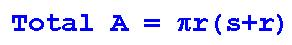HOME

This page shows you how to use the formula for the Total Area of a Cone.

Instructions:

Click on the Example sheet, the Exercise sheet and the Answers sheet in order to view and print them.

Watch the video as many times as you wish.

Use the Example sheet as a model to help you complete the Exercise sheet.

Use the Answers sheet to correct your work.

Video #1: Examples 1, 2 and 3:

Video #2: Examples 4 and 5:

Video #3: Why We Don't Have To Memorize The Formula For Total Area Of A Cone:

Here is the formula for Total Area of a cone: